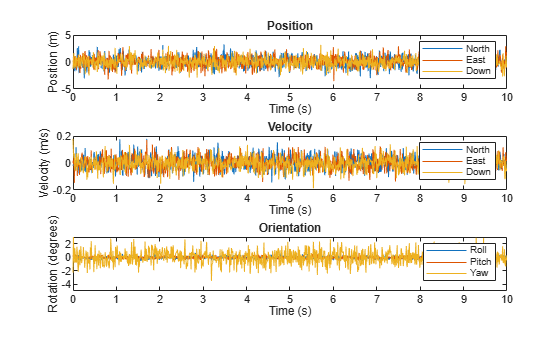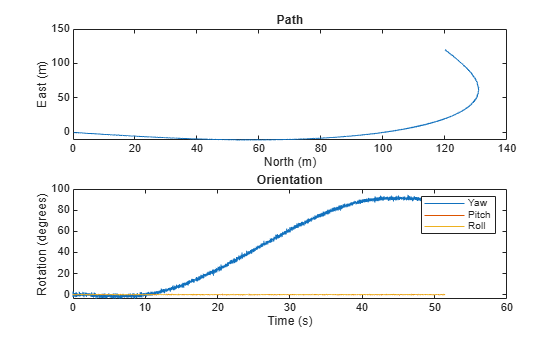# insSensor

Inertial navigation system and GNSS/GPS simulation model

## Description

The `insSensor` System object™ models a device that fuses measurements from an inertial navigation system (INS) and global navigation satellite system (GNSS) such as a GPS, and outputs the fused measurements.

To output fused INS and GNSS measurements:

1. Create the `insSensor` object and set its properties.

2. Call the object with arguments, as if it were a function.

## Creation

### Syntax

``INS = insSensor``
``INS = insSensor(Name,Value)``

### Description

example

````INS = insSensor` returns a System object, `INS`, that models a device that outputs measurements from an INS and GNSS.```

example

````INS = insSensor(Name,Value)` sets properties using one or more name-value pairs. Unspecified properties have default values. Enclose each property name in quotes.```

## Properties

expand all

Unless otherwise indicated, properties are nontunable, which means you cannot change their values after calling the object. Objects lock when you call them, and the `release` function unlocks them.

If a property is tunable, you can change its value at any time.

Location of the sensor on the platform, in meters, specified as a three-element real-valued vector of the form [x y z]. The vector defines the offset of the sensor origin from the origin of the platform.

Tunable: Yes

Data Types: `single` | `double`

Accuracy of the roll measurement of the sensor body, in degrees, specified as a nonnegative real scalar.

Roll is the rotation around the x-axis of the sensor body. Roll noise is modeled as a white noise process. `RollAccuracy` sets the standard deviation of the roll measurement noise.

Tunable: Yes

Data Types: `single` | `double`

Accuracy of the pitch measurement of the sensor body, in degrees, specified as a nonnegative real scalar.

Pitch is the rotation around the y-axis of the sensor body. Pitch noise is modeled as a white noise process. `PitchAccuracy` defines the standard deviation of the pitch measurement noise.

Tunable: Yes

Data Types: `single` | `double`

Accuracy of the yaw measurement of the sensor body, in degrees, specified as a nonnegative real scalar.

Yaw is the rotation around the z-axis of the sensor body. Yaw noise is modeled as a white noise process. `YawAccuracy` defines the standard deviation of the yaw measurement noise.

Tunable: Yes

Data Types: `single` | `double`

Accuracy of the position measurement of the sensor body, in meters, specified as a nonnegative real scalar or a three-element real-valued vector. The elements of the vector set the accuracy of the x-, y-, and z-position measurements, respectively. If you specify `PositionAccuracy` as a scalar value, then the object sets the accuracy of all three positions to this value.

Position noise is modeled as a white noise process. `PositionAccuracy` defines the standard deviation of the position measurement noise.

Tunable: Yes

Data Types: `single` | `double`

Accuracy of the velocity measurement of the sensor body, in meters per second, specified as a nonnegative real scalar.

Velocity noise is modeled as a white noise process. `VelocityAccuracy` defines the standard deviation of the velocity measurement noise.

Tunable: Yes

Data Types: `single` | `double`

Accuracy of the acceleration measurement of the sensor body, in meters per second, specified as a nonnegative real scalar.

Acceleration noise is modeled as a white noise process. `AccelerationAccuracy` defines the standard deviation of the acceleration measurement noise.

Tunable: Yes

Data Types: `single` | `double`

Accuracy of the angular velocity measurement of the sensor body, in meters per second, specified as a nonnegative real scalar.

Angular velocity is modeled as a white noise process. `AngularVelocityAccuracy` defines the standard deviation of the acceleration measurement noise.

Tunable: Yes

Data Types: `single` | `double`

Enable input of simulation time, specified as a logical `0` (`false`) or `1` (`true`). Set this property to `true` to input the simulation time by using the `simTime` argument.

Tunable: No

Data Types: `logical`

Enable GNSS fix, specified as a logical `1` (`true`) or `0` (`false`). Set this property to `false` to simulate the loss of a GNSS receiver fix. When a GNSS receiver fix is lost, position measurements drift at a rate specified by the `PositionErrorFactor` property.

Tunable: Yes

#### Dependencies

To enable this property, set `TimeInput` to `true`.

Data Types: `logical`

Position error factor without GNSS fix, specified as a scalar or a 1-by-3 vector of scalars.

When the `HasGNSSFix` property is set to `false`, the position error grows at a quadratic rate due to constant bias in the accelerometer. The position error for a position component E(t) can be expressed as E(t) = 1/2αt2, where α is the position error factor for the corresponding component and t is the time since the GNSS fix is lost. While running, the object computes t based on the `simTime` input. The computed E(t) values for the x, y, and z components are added to the corresponding position components of the `gTruth` input.

Tunable: Yes

#### Dependencies

To enable this property, set `TimeInput` to `true` and `HasGNSSFix` to `false`.

Data Types: `single` | `double`

Random number source, specified as one of these options:

• `'Global stream'` –– Generate random numbers using the current global random number stream.

• `'mt19937ar with seed'` –– Generate random numbers using the mt19937ar algorithm, with the seed specified by the `Seed` property.

Data Types: `char` | `string`

Initial seed of the mt19937ar random number generator algorithm, specified as a nonnegative integer.

#### Dependencies

To enable this property, set `RandomStream` to `'mt19937ar with seed'`.

Data Types: `single` | `double` | `int8` | `int16` | `int32` | `int64` | `uint8` | `uint16` | `uint32` | `uint64`

## Usage

### Syntax

``measurement = INS(gTruth)``
``measurement = INS(gTruth,simTime)``

### Description

example

````measurement = INS(gTruth)` models the data received from an INS sensor reading and GNSS sensor reading. The output measurement is based on the inertial ground-truth state of the sensor body, `gTruth`.```
````measurement = INS(gTruth,simTime)` additionally specifies the time of simulation, `simTime`. To enable this syntax, set the `TimeInput` property to `true`.```

### Input Arguments

expand all

Inertial ground-truth state of sensor body, in local Cartesian coordinates, specified as a structure containing these fields:

FieldDescription
`'Position'`

Position, in meters, specified as a real, finite N-by-3 matrix of [x y z] vectors. N is the number of samples in the current frame.

`'Velocity'`

Velocity (v), in meters per second, specified as a real, finite N-by-3 matrix of [vx vy vz] vector. N is the number of samples in the current frame.

`'Orientation'`

Orientation with respect to the local Cartesian coordinate system, specified as one of these options:

• N-element column vector of `quaternion` objects

• 3-by-3-by-N array of rotation matrices

• N-by-3 matrix of [xroll ypitch zyaw] angles in degrees

Each quaternion or rotation matrix is a frame rotation from the local Cartesian coordinate system to the current sensor body coordinate system. N is the number of samples in the current frame.

`'Acceleration'`

Acceleration (a), in meters per second squared, specified as a real, finite N-by-3 matrix of [ax ay az] vectors. N is the number of samples in the current frame.

`'AngularVelocity'`

Angular velocity (ω), in meters per second squared, specified as a real, finite N-by-3 matrix of [ωx ωy ωz] vectors. N is the number of samples in the current frame.

The field values must be of type `double` or `single`.

The `Position`, `Velocity`, and `Orientation` fields are required. The other fields are optional.

Example: ```struct('Position',[0 0 0],'Velocity',[0 0 0],'Orientation',quaternion([1 0 0 0]))```

Simulation time, in seconds, specified as a nonnegative real scalar.

Data Types: `single` | `double`

### Output Arguments

expand all

Measurement of the sensor body motion, in local Cartesian coordinates, returned as a structure containing these fields:

FieldDescription
`'Position'`

Position, in meters, specified as a real, finite N-by-3 matrix of [x y z] vectors. N is the number of samples in the current frame.

`'Velocity'`

Velocity (v), in meters per second, specified as a real, finite N-by-3 matrix of [vx vy vz] vector. N is the number of samples in the current frame.

`'Orientation'`

Orientation with respect to the local Cartesian coordinate system, specified as one of these options:

• N-element column vector of `quaternion` objects

• 3-by-3-by-N array of rotation matrices

• N-by-3 matrix of [xroll ypitch zyaw] angles in degrees

Each quaternion or rotation matrix is a frame rotation from the local Cartesian coordinate system to the current sensor body coordinate system. N is the number of samples in the current frame.

`'Acceleration'`

Acceleration (a), in meters per second squared, specified as a real, finite N-by-3 matrix of [ax ay az] vectors. N is the number of samples in the current frame.

`'AngularVelocity'`

Angular velocity (ω), in meters per second squared, specified as a real, finite N-by-3 matrix of [ωx ωy ωz] vectors. N is the number of samples in the current frame.

The returned field values are of type `double` or `single` and are of the same type as the corresponding field values in the `gTruth` input.

## Object Functions

To use an object function, specify the System object as the first input argument. For example, to release system resources of a System object named `obj`, use this syntax:

`release(obj)`

expand all

 `perturbations` Perturbation defined on object `perturb` Apply perturbations to object
 `step` Run System object algorithm `clone` Create duplicate System object `isLocked` Determine if System object is in use `reset` Reset internal states of System object `release` Release resources and allow changes to System object property values and input characteristics

## Examples

collapse all

Create a motion structure that defines a stationary position at the local north-east-down (NED) origin. Because the platform is stationary, you need to define only a single sample. Assume the ground-truth motion is sampled for 10 seconds with a 100 Hz sample rate. Create a default `insSensor` System object™. Preallocate variables to hold output from the `insSensor` object.

```Fs = 100; duration = 10; numSamples = Fs*duration; motion = struct( ... 'Position',zeros(1,3), ... 'Velocity',zeros(1,3), ... 'Orientation',ones(1,1,'quaternion')); INS = insSensor; positionMeasurements = zeros(numSamples,3); velocityMeasurements = zeros(numSamples,3); orientationMeasurements = zeros(numSamples,1,'quaternion');```

In a loop, call `INS` with the stationary motion structure to return the position, velocity, and orientation measurements in the local NED coordinate system. Log the position, velocity, and orientation measurements.

```for i = 1:numSamples measurements = INS(motion); positionMeasurements(i,:) = measurements.Position; velocityMeasurements(i,:) = measurements.Velocity; orientationMeasurements(i) = measurements.Orientation; end```

Convert the orientation from quaternions to Euler angles for visualization purposes. Plot the position, velocity, and orientation measurements over time.

```orientationMeasurements = eulerd(orientationMeasurements,'ZYX','frame'); t = (0:(numSamples-1))/Fs; subplot(3,1,1) plot(t,positionMeasurements) title('Position') xlabel('Time (s)') ylabel('Position (m)') legend('North','East','Down') subplot(3,1,2) plot(t,velocityMeasurements) title('Velocity') xlabel('Time (s)') ylabel('Velocity (m/s)') legend('North','East','Down') subplot(3,1,3) plot(t,orientationMeasurements) title('Orientation') xlabel('Time (s)') ylabel('Rotation (degrees)') legend('Roll', 'Pitch', 'Yaw')```Generate INS measurements using the `insSensor` System object™. Use `waypointTrajectory` to generate the ground-truth path.

Specify a ground-truth orientation that begins with the sensor body x-axis aligned with North and ends with the sensor body x-axis aligned with East. Specify waypoints for an arc trajectory and a time-of-arrival vector for the corresponding waypoints. Use a 100 Hz sample rate. Create a `waypointTrajectory` System object with the waypoint constraints, and set `SamplesPerFrame` so that the entire trajectory is output with one call.

```eulerAngles = [0,0,0; ... 0,0,0; ... 90,0,0; ... 90,0,0]; orientation = quaternion(eulerAngles,'eulerd','ZYX','frame'); r = 20; waypoints = [0,0,0; ... 100,0,0; ... 100+r,r,0; ... 100+r,100+r,0]; toa = [0,10,10+(2*pi*r/4),20+(2*pi*r/4)]; Fs = 100; numSamples = floor(Fs*toa(end)); path = waypointTrajectory('Waypoints',waypoints, ... 'TimeOfArrival',toa, ... 'Orientation',orientation, ... 'SampleRate',Fs, ... 'SamplesPerFrame',numSamples);```

Create an `insSensor` System object to model receiving INS data. Set the `PositionAccuracy` to `0.1`.

`ins = insSensor('PositionAccuracy',0.1);`

Call the waypoint trajectory object, `path`, to generate the ground-truth motion. Call the INS simulator, `ins`, with the ground-truth motion to generate INS measurements.

```[motion.Position,motion.Orientation,motion.Velocity] = path(); insMeas = ins(motion);```

Convert the orientation returned by `ins` to Euler angles in degrees for visualization purposes. Plot the full path and orientation over time.

```orientationMeasurementEuler = eulerd(insMeas.Orientation,'ZYX','frame'); subplot(2,1,1) plot(insMeas.Position(:,1),insMeas.Position(:,2)); title('Path') xlabel('North (m)') ylabel('East (m)') subplot(2,1,2) t = (0:(numSamples-1)).'/Fs; plot(t,orientationMeasurementEuler(:,1), ... t,orientationMeasurementEuler(:,2), ... t,orientationMeasurementEuler(:,3)); title('Orientation') legend('Yaw','Pitch','Roll') xlabel('Time (s)') ylabel('Rotation (degrees)')```## Extended Capabilities

### Objects

Introduced in R2020b

## SupportGet trial now